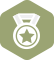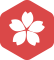### pyhon如何生成100行2列的随机数GitHub 绑定GitHub第三方账户获取2020年1月 其他开发语言大版内专家分月排行榜第一
2019年12月 其他开发语言大版内专家分月排行榜第一
2019年7月 其他开发语言大版内专家分月排行榜第一
2019年4月 其他开发语言大版内专家分月排行榜第一
2019年3月 其他开发语言大版内专家分月排行榜第一
2019年1月 其他开发语言大版内专家分月排行榜第一tiger波波

Python里面如何生成随机数

Python中的random模块用于生成随机数。下面介绍一下random模块中最常用的几个函数。   random.random random.random()用于生成一个0到1的随机符点数: 0 random.uniform 　random.uniform的函数原型为...

python 生成随机矩阵和随机数

Python 生成一组随机数列表

python 生成随机数的三种方法

1. 使用 random 包生成随机数 可以生成 均匀分布， 高斯分布，（包括正态分布） 指数分布，（与泊松分布有区别：泊松分布表示一段时间发生多少次，而指数分布表示两次发生的时间间隔） 贝塔分布， 韦布尔分布的...

Python】numpy中矩阵和随机数生成

np.array(range(10)) # array([0, 1, 2, 3, 4, 5, 6, 7, 8, 9]) np.array(range(5,10,2)) # range最多3个参数，第1个起始；第2个结束（不包括）；第3个为步长 np.arange(10) # array([0, 1, 2, 3, ...

python 随机产生0-100以内的随机数，找到最大值和最小值并交换位置

import random #随机模块 number = [] #创建一个列表 for i in range(0,100): #循环随机数100位 num = random.randint(0,100) ...

python如何一次性取出多个随机数

Python内置的函数一次只能生成一个随机数，然而你可以方便地使用表理解(list comprehension)一次性生成多个随机数。示例的代码如下： import random [random.randint(0,100) for _ in range(10)] #[57

Python3：在0-1之间生成10个随机数

Python生成任意范围的固定随机数

import numpy as np random = np.random.RandomState(0) #随机数种子，相同种子下每次运行生成随机数相同 color = random.uniform(0., 1., size=3)

Python生成不重复的随机数

random.sample(range(1,N), k) 表示从[1,N]的范围内随机生成k个数，结果以列表返回 栗子1： 随机生成5个(1, 10)范围内的数字： import random for i in range(5): L1 = random.randint(1, 10)...栗子2： 随机生成5...

python生成n个指定范围内的随机数

python生成n个指定范围内的随机数 ①输入随机数范围和个数 ②判断随机数个数是否小于n ③随机生成一个随机数 ④判断随机数是否在列表中存在 ⑤如果不存在，则将该随机数存进列表 重复步骤②到⑤ 看代码： import ...

python生成一组随机数

python代码生成服从正态分布的随机数

1）生成随机数 import numpy as np #导入库 random3 = numpy.random.randn(10000) #随机生成10000个服从正态分布的随机数 2）结果验证 import seaborn as sn...

Python 生成正态随机数

Python 生成正态随机数import numpy as np from numpy.linalg import cholesky import matplotlib.pyplot as pltsampleNo = 1000; mu = 85 sigma = 4 np.random.seed(0) s = np.random.normal(mu, sigma, sampleNo )...

Python2.7——生成任意范围任意精度的随机数

# -*- coding: utf-8 -*- ...random = np.random.RandomState(0)#RandomState生成随机数种子 for i in range(200):#随机数个数 a = random.uniform(-0.1, 0.1)#随机数范围 print round(a, 2)#随机数精度要求

Python生成10个0-100之间的随机数，将其每个数一写入“随机数.txt”文件，然后从文件中读出十个数，排序...

import random i = 0 f = open(r'E:\Python\随机数.txt','w+') while i < 10: y = int(random.random()*100) print(y) f.write(str(y)+"\n") f.flush() i = i + 1 li = [] with op...

Python利用random生成一个列表内的随机数

[python]产生10个随机数

Python 输出10个不重复的随机数_范围10-100

import random h =set() while(len(h)&lt;10): h.add(random.randint(10,100)) print(h)

Python随机数种子的作用及使用

1.1随机数种子的作用 随机数种子（random seed)在伪随机数生成器中用于生成伪随机数的初始数值。对于一个伪随机数生成器，从相同的随机数种子出发，可以得到相同的随机数序列。随机数种子通常由当前计算机状态确定...

python产生100随机数,每个随机数之间的差值为固定值?

Python生成一定范围内指定数目的无重复随机数

python在指定范围内产生n个不重复的随机数的两种办法

#第一个参数表示开始的范围，第个参数表示结束的范围，第三个表示产生随机数的个数 #最后返回的是一个列表 import random class CreateRandomPage: def __init__(self, begin, end, needcount): ...

python随机数产生100个整数(0-100),统计出现次数最多的数字.

import random # 随机数产生100个整数(0-100),放入一个列表中,统计出现次数最多的数字. # 1.存放随机数列表 ...生成随机数 num = random.randint(0,100) # 4.添加到列表中 number.append(num) pr...

python实现指定长度的随机数

import random def random_20char(string,length): for i in range(length): x = random.randint(1,2) if x == 1: y = str(random.randint(0,9)) else: y = ch...

python生成随机整数，随机小数，0-1之间的小数

python生成随机整数，随机小数，0-1之间的小数 1.生成随机整数 import random print(random.randint(1,100))#生成1到100之间的随机整数 2.生成0-1之间随机的小数 import numpy as np print(random....

Python 产生特定范围内不重复多个随机数的两种方法

Python生成随机数的几种方法

Python中用于生成随机数的模块是random，在使用前需要import。 random.random():生成一个0-1之间的随机浮点数 random.randint(a,b):生成[a,b]之间的整数,包含a,b random.uniform(a,b):生成[a,b]之间的浮点数 ...

Python 里面如何生成随机数？（random）

Python 中用于生成随机数的模块是 random，在使用前需要 import. 如下例子可以酌情 举： random.random()：生成一个 0-1 之间的随机浮点数； random.uniform(a, b)：生成[a,b]之间的浮点数； random.randint(a,...

python随机数种子seed()的讲解

python的random.seed()随机数的使用 import random random.seed( 0 ) print("Random number with seed 10 : ", random.random()) #生成同一个随机数 random.seed(0) print("Random number with seed 10 : ", random....